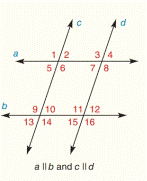Chapter 2.CR, Problem 3CR### Elementary Geometry for College St...

6th Edition
Daniel C. Alexander + 1 other
ISBN: 9781285195698

#### Solutions

Chapter
Section### Elementary Geometry for College St...

6th Edition
Daniel C. Alexander + 1 other
ISBN: 9781285195698
Textbook Problem
1 views

# Given: m ∠ 9 = 2 x + 17 m ∠ 11 = 5 x − 94 Find: xTo determine

To find:

The value of x.

Explanation

Given:

The given statement is,

m9=2x+17m11=5x94abcd

Figure (1)

Property:

If two parallel lines are cut by a transversal, then a pair of corresponding angles is congruent.

Calculation:

The given statement is,

m9=2x+17m11=5x94abcd

9 and 11 are corresponding angles

### Still sussing out bartleby?

Check out a sample textbook solution.

See a sample solution

#### The Solution to Your Study Problems

Bartleby provides explanations to thousands of textbook problems written by our experts, many with advanced degrees!

Get Started